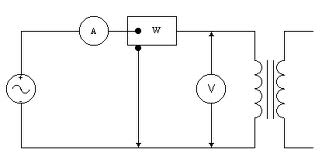## Open Circuit Test and Short Circuit Test Assignment Help

Assignment Help: >> Transformer - Open Circuit Test and Short Circuit Test

Open Circuit Test and Short Circuit Test:

We should know the losses of a transformer to find out its efficiency. We will discuss the two main losses in the transformer given below.Copper Losses or Ohmic Losses (I2 R -loss)

These losses occur in the primary and secondary winding resistances. These losses vary as the square of the current (loading).

Core Loss or Iron Loss

Core loss comprises eddy current loss and hysteresis loss resulting from alternations of magnetic flux in the core.

Pe = Eddy current losses = Ke f2 B2 W/M3

and      Ph = Hysteresis losses = Kh f B1.6 W/M3

where Ke and Kh are constants.

f → Supply frequency, Hz

B → Flux density, Wb/M2

The eddy current losses may be minimised by using the laminated core steel and the hysteresis losses may be minimised by adding silicon content to the iron core.

Open circuit test and short circuit tests are performed to find out the iron losses and copper losses respectively.

 Open Circuit Test Short Circuit Test